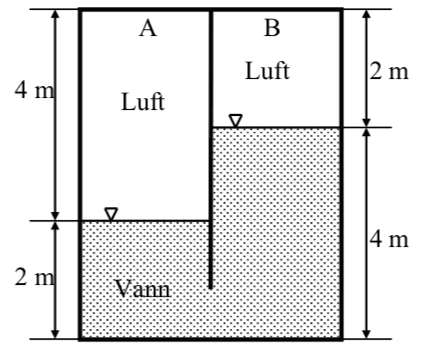# Pressure at same height in same fluid?

## Homework Statement

A sealed has a partition stuck down in water, creating two separate air pockets. The pressure at A is measured to 95 kPa (absolute) and the temperature is 20 C. Find the pressure at B.## The Attempt at a Solution

Why is the pressure at A not equal to B? Doing U-tube exercises one uses that the pressure at the same height in the same fluid is equal, which is not the case here. Is it because the tank is sealed?

#### Attachments

If I'm not mistaken:
Yes, it's because the tank is sealed. The water is at different heights so the pressure differential is given by:
$$\rho_A g h_1 - \rho_w g h_2 - \rho_B g h_3 \\ = g(2\rho_A - 2\rho_w) \\ = 2g(\rho_A-\rho_w)$$
assuming ##\rho_A = \rho_B##

its been a while since i've done fluids so i may indeed be mistaken, take it with a grain of salt :)

CWatters
Homework Helper
Gold Member
Yes.

When we think about the pressure under water we sometimes ignore the effect of the air above because it's roughly the same everywhere but that's not always valid. Changes in the air pressure above the water changes the pressure below the water. This can cause the sea to rise and fall in different places (an example is a storm surge).

Consider the pressure at the bottom of the tank. It must be the same pressure on the left as the right or fluid would flow horizontally. However the depth of water on the right is greater than that on the left by 2m. So something must be equalising the pressure at the bottom OK?

Imagine if the tank was open at the top. The water on the right would fall until it was equal both sides. So something else must be preventing this and equalising the pressure at the bottom. One way to think of it is that the water has already fallen a bit on the right and risen on the left. This increased air pressure on the left and reduced pressure on the right is what causes the pressure at the bottom to be equal.

Thanks! is it possible to prove that the pressure at the bottom of the tank HAVE to equal each other?

The fluid is static. ie. in equilibrium. Remember that you can think of pressure at a point as being exerted equally onto that point from all directions. So if the pressures were different at the bottom on the left and right, then there would be a net flow from high pressure to low pressure ie not static

•CWatters